# KSEEB Solutions for Class 6 Maths Chapter 10 Mensuration Ex 10.1

Students can Download Chapter 10 Mensuration Ex 10.1 Questions and Answers, Notes Pdf, KSEEB Solutions for Class 6 Maths helps you to revise the complete Karnataka State Board Syllabus and score more marks in your examinations.

## Karnataka State Syllabus Class 6 Maths Chapter 10 Mensuration Ex 10.1

Question 1.
Find the perimeter of each of the following figures: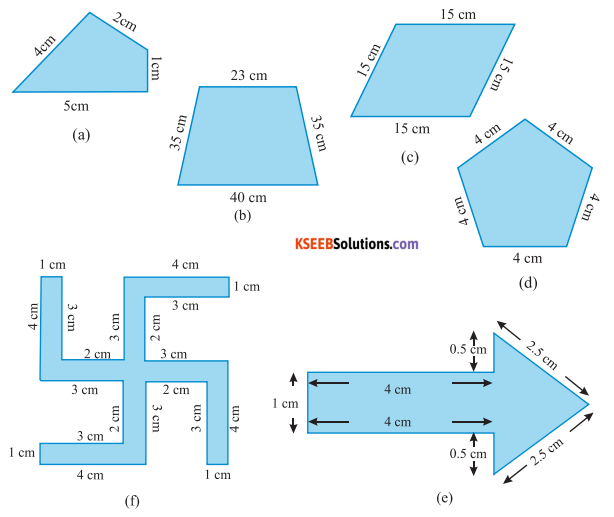Solution :
Perimeter of a polygon is equal to the sum of the lengths of all sides of that polygon.
(a) Perimeter = (4 + 2 +1 + 5) cm = 12 cm
(b) Perimeter = (23 + 35 + 40 + 35) cm = 133 cm
(c) Perimeter = (15 + 15 + 15 + 15) cm = 60 cm
(d) Perimeter = (4 + 4 + 4 + 4 +4) cm = 20 cm
(e) Perimeter = (1 + 4 + 0.5 + 2.5 + 2.5 + 0.5+ 4) cm = 15 cm
(f) Perimeter = (1 + 3 + 2 + 3 + 4 + 1 + 3 + 2 + 3 + 4 + 1 + 3 + 2 + 3 + 4 + 1 + 3 + 2 + 3 + 4) = 52 cm

Question 2.
The lid of a rectangular box of sides 40 cm by 10 cm is sealed all round with tape. What is the length of the tape required?
Solution:
Length (l) of rectangular box = 40 cm
Breadth (b) of rectangular box = 10 cm
Length o f tape required = perimeter of rectangular box
= 2 ( l + b)
= 2(40 + 10) = 100 cmQuestion 3.
A table – top measures 2 m 25 cm by 1 m 50 cm. What is the perimeter of the table – top?
Solution:
Length (l) of the top of the table = 2 m 25 cm = 2 + 0.25
= 2.25 m
Breadth (b) of the top of the table = 1 m 50 cm = 1 + 0.50 = 1.50 m
Perimeter of table – top = 2 (l + b)
= 2 × (2.25 + 1.50)
= 2 × 3.75 = 7.5 m
The perimeter of the table-top is 7.5 m

Question 4.
What is the length of the wooden strip required to frame a photograph of length and breadth 32 cm and 21 cm respectively?
Solution:
Length (l) of the photograph = 32cm
Breadth (b) of the photograph = 21 cm
Perimeter of photograph = 2 × (l + b)
= 2 × (32 + 21)
= 2 × (53)
= 106 cm
Length of wooden strip required is 106 cm.Question 5.
A rectangular piece of land measures 0.7 km by 0.5 km. Each side is to be fenced with 4 rows of wires. What is the length of the wire needed?
Solution:
Given,
Length of the Land (l) = 0.7 km
Breadth of the land (b) = 0.5 km.
perimeter of the land = 2 × (l + b)
= 2 × (0.7 + 0.5)
= 2 × (1.2)
= 2.4 km.
The length of the wire required to fenced the land is given by 4 × 2.4 = 9.6 km.

Question 6.
Find the perimeter of each of the following Shapes:
a) A triangle of sides 3 cm, 4 cm and 5 cm.
b) An equilateral triangle of side 9 cm.
c) An isosceles triangle with equal sides 8 cm each and third side 6 cm.
Solution:
a) perimeter of a ∆le = (AB + BC + CA) cm
= (3 + 4 + 5) cm.
= 12 cm

b) perimeter of an equilateral triangle = 3 × side of ∆le
= (3 × 9 ) cm
= 27 cm.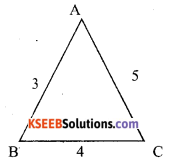c) perimeter of an isosceles triangle = 8 + 8 + 6 = 22 cm.Question 7.
Find the perimeter of a triangle with sides measuring 10 cm, 14 cm, and 15 cm.
Solution:
Perimeter of triangle = sum of lengths of all sides of the triangle
∴ Perimeter of ∆le = 10 + 14 + 15 = 39 cm.

Question 8.
Find the perimeter of a regular hexagon with each side measuring 8m.
Solution:
Perimeter of regular hexagon = 6 × side of regular hexagon
= 6 × 8 m
∴ Perimeter of regular hexagon = 48 m

Question 9.
Find the side of the square whose perimeter is 20m.
Solution:
Perimeter of square = 4 × side 20
= 4 × side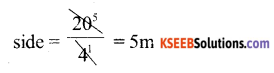∴ Side of the square = 5 mQuestion 10.
The perimeter of a regular pentagon is 100 cm. How long is its each side?
Solution:
Perimeter of regular pentagon = 5 × side of regular pentagon.
100 = 5 × side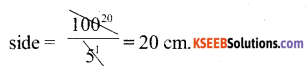∴ Side of the regular pentagon = 20 cm.

Question 11.
A piece of string is 30 cm long. What will be the length of each side if the string is used to form:
a) a square?
b) an equilateral triangle?
c) a regular hexagon?
Solution:
a) perimeter a square = 4 × side of the square
= 30 = 4 × side of the square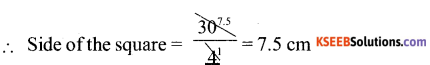Side of the square = 7.5 cm.

b) perimeter an equilateral triangle = 3 × side of the ∆le
30 = 3 × side of the ∆le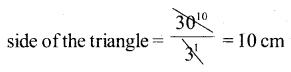Side of the equilateral ∆le = 10 cm.

c) perimeter of a regular hexagon = 6 × side of the regular hexagon
30 = 6 × side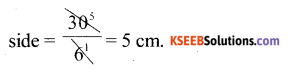Side of the regular hexagon = 5 cm.Question 12.
Two sides of a triangle are 12 cm and 14 cm. The perimeter of the triangle is 36 cm. What is its third side?
Solution:
Given AB = 12 cm, and BC = 14 cm, CA = ?
Perimeter of triangle = sum of all sides of the triangles
36 = AB + BC + CA
36 = 26 + CA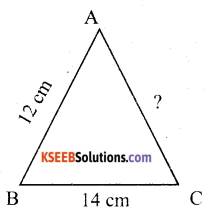CA = 36 – 26 = 10 cm.
CA = 10 cm.
Hence, the third side of the triangle is 10 cm.

Question 13.
Find the cost of fencing a square park of side 250 m at the rate of Rs 20 per metre.
Solution:
Length of fence required is equal to the perimeter of the square park
= 4 × side
= 4 × 250
= 1000 m
Cost for fencing 1 m of square park = Rs. 20
Cost for fencing 1000m of square park = 1000 × 20
= Rs. 20,000Question 14.
Find the cost of fencing a rectangular park of length 175 m and breadth 125 m at the rate of Rs 12 per metre.
Solution:
Given:-
Length of rectangular park (l) = 175 cm
Breadth of rectangular park (b) = 125 cm
Length of wire required for fencing the park = Perimeter of the park
= 2 × (l + b)
= 2 × (175 + 125)
= 2 × 300 = 600 m
Cost for fencing 1 m of the park = Rs 12.
Cost for fencing 600m of the square park = 600 × 12
= Rs. 7200

Question 15.
Sweety runs around a square park of side 75m. Bulbul runs around a rectangular park with length 60m and breadth 45m. Who covers less distance?
Solution:
The distance covered by sweety = 4 × rectangular square park
= 4 × 75 m = 300 m
The distance covered by bulbul = perimeter of rectangular park
= 2 × (60 + 45)
= 2 × 105
= 210 m
Therefore, bulbul covers the less distance than sweety.Question 16.
What is the perimeter of each of the following figures? What do you infer from the answers?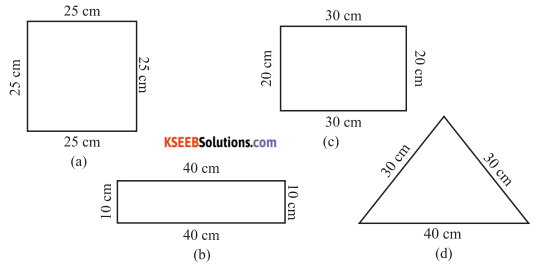Solution:
a) perimeter of square = 4 × 25 = 100 cm.
b) perimeter of rectangle = 2 × (10 + 40) = 2 × 50 = 100 cm
c) perimeter of rectangle = 2 × (20 + 30) = 100 cm.
d) perimeter of ∆le = 30 + 30 + 40 = 100 cm
Imfered:- The above figures have the same perimetre .

Question 17.
Avneet buys 9 square paving slabs, each with a side of $$\frac{1}{2}$$ m. He lays them in the form of a square.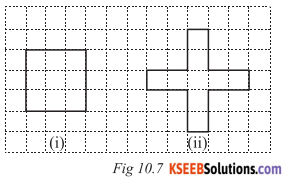Solution:
a) What iis the perimeter of his arrangement [ Fig 10.7 (i)] ?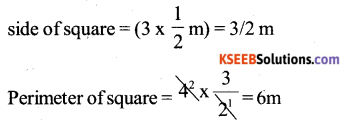b) Shari does not like his arrangement. She gets him to lay them out like a cross. What is the perimeter of her arrangement [(Fig 10.7(ii)]?
perimeter of cross = 0.5 + 1 + 1 + 0.5 + 1 + 1 + 0.5 + 1 + 1 + 0.5 + 1 + 1
= 10 m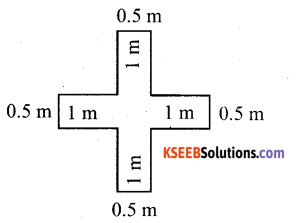c) Which has greater perimeter?
The arrangement in the form of a cross has a greater perimeter.

d) Avneet wonders if there is a way of getting an even greater perimeter. Can you find a way of doing this? (The paving slabs must meet along complete edges i.e. they cannot be broken?
Arrangements with perimeter greater than 10m can not be determined.